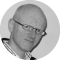Screenwriter, Choreographer, Game Developer

# Simple example of acceleration

Posted on

While teaching my Actionscript Fundamentals course a couple of days ago one of the trainees asked me how to do a simple example of using acceleration and deceleration within an animation. Here it is. Roll over the centre of the propeller below.

The code looks like this.

```var rate:Number = 0;
var acceleration:Number = 0.5;

prop_mc.centre_mc.onRollOver = function()
{
prop_mc.onEnterFrame = function()
{
rate += acceleration;
rate = Math.min( rate, 15 );
prop_mc._rotation += rate;
}
};

prop_mc.centre_mc.onRollOut = function()
{
prop_mc.onEnterFrame = function()
{
rate -= acceleration;
rate = Math.max( rate, 0 );
prop_mc._rotation += rate;
}
};

prop_mc.centre_mc.onDragOver = prop_mc.centre_mc.onRollOver;
prop_mc.centre_mc.onDragOut = prop_mc.centre_mc.onRollOut;```

For a brief explaination, rate is the speed of rotation of the propeller. When the mouse is over the propeller, the rate increases each frame by the acceleration and is capped at a maximum rate of 15. When the mouse is not over the propeller, the rate decreases each frame by the acceleration and is capped at zero. The result is what you see.